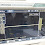# DIY Mini 12Volt Power Amplifier

On this occasion, I will share a mini power amplifier with a power output that is not big enough, and this power amplifier can be supplied with 12volt voltage up to 24V asymmetric. With a small power, this amplifier can be applied for home use or on a radio or portable speakers. For circuit schematics and PCB layout my own design as shown below.

Component list:
R1=560K, R2=22R, R3=6K8, R4=6K8, R5=2K2, R6=1K, R7=22K, R8=1R, R9=1R, R10=15R, R11=150R RV1=10K-100K Potentiometer
C1=10uF, C2= 10uF, C3=330pF, C4=220uF, C5=100uF, C6=330uF
Q1=C945/ C828, Q2=BD139, Q3=TIP32, Q4=TIP31
D1=1N4007/ 1N4148

PCB Layout Design:
Finished Project:
Full video making of this 12Volt Power Amplifier circuit by using PC power supply former, the input voltage about 14V DC asymmetric with current output 5A-20A. For the final transistor is using D1763 and B1186 you can also using TIP31/TIP32. The speaker using a 2x2,5inch speaker with maximum power 60W, see below the video:

## 9 comments for "DIY Mini 12Volt Power Amplifier"

1.1.2.Why Q3 is emitter connected to ground? Is it a push-pull scheme? It does and does not look like this.

3.what is the voltage for all capacitors ?

4.Can I use tip 41/42 instead of tip 32/31?

5.Love all you guys like family

6.7.8.Alguien a construido el circuito